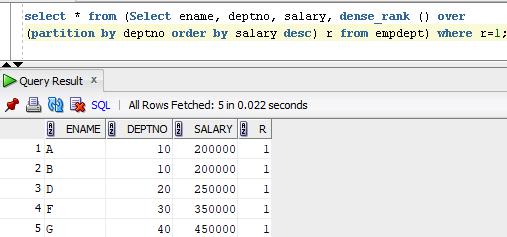# Difference between Rank and Dense_Rank Functions and Nth Highest salary

Jul 30, 2019## Rank ():

RANK function is used to rank the repeating values in a manner such that similar values are ranked the same. In other words, rank function returns the rank of each row within the partition of a result set. The rank of a row is one plus the number of ranks that come before the row in question. The RANK () function is also useful to find the top-N and bottom-N reports.

## Partition_by_clause:

This is used to splits the rows generated into different sets. If partition_by_clause is not specified, then all rows shall be treated as a single group.

## Order_By_Clause:

This is used to sort the Partitioned data into specified order.

#### Example for Rank Function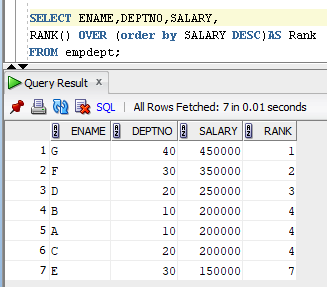In the above example we can see the value of salary 20000 is repeated for 3 times and we have same rank number 4 is repeated for 3 times and next rank value is 7.

#### Example for RANK function with partition: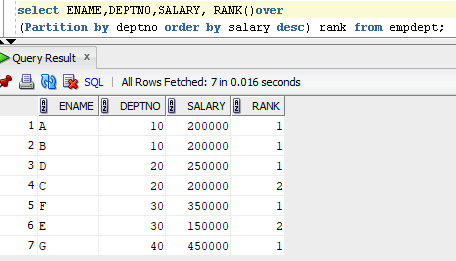In the above Output we can see the rank is partitioned (Grouped) by DEPTNO.

## DENSE_RANK ():

The DENSE_RANK () will assign the rank number to each record present in a partition without skipping the rank numbers. dense_rank function returns the rank of each row in continuous series within the partition of a result set. The rank of a row is one plus the number of distinct ranks that come before the row in question.

## Syntax:

SELECT DENSE_RANK () OVER (PARTITION_BY_Clause ORDER_BY_Clause) FROM [Source]

## Partition_by_clause:

This is used to splits the rows generated into different sets. If partition_by_clause is not specified, then all rows shall be treated as a single group.

## Order_By_Clause:

This is used to sort the Partitioned data into specified order.

#### Example for DENSE_RANK Function: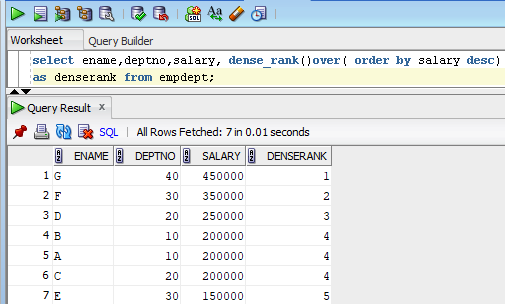In the above example we can see the value of salary 20000 is repeated for 3 times and we have same rank number 4 is repeated for 3 times and next rank value is 5.

#### Example for DENSE_RANK function with partition: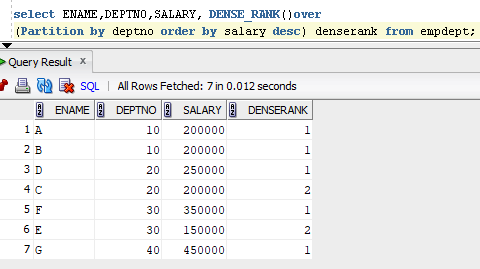For DENSE_RANK function the rank is partitioned(Grouped) by DEPTNO.

## Query to find the Nth Highest Salary in the table:

In order to find nth highest salary in the table we use RANK () or DENSE_RANK () based on requirement.

## Below is the sample table data with ranks:## To find the 3rd highest salary below is the Query:

### (order by salary desc) r from empdept) where r=3;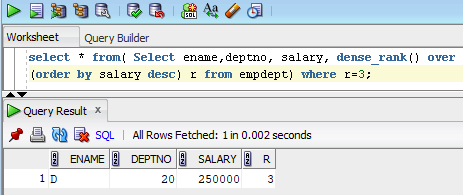To find the 1st highest salary Department number wise: